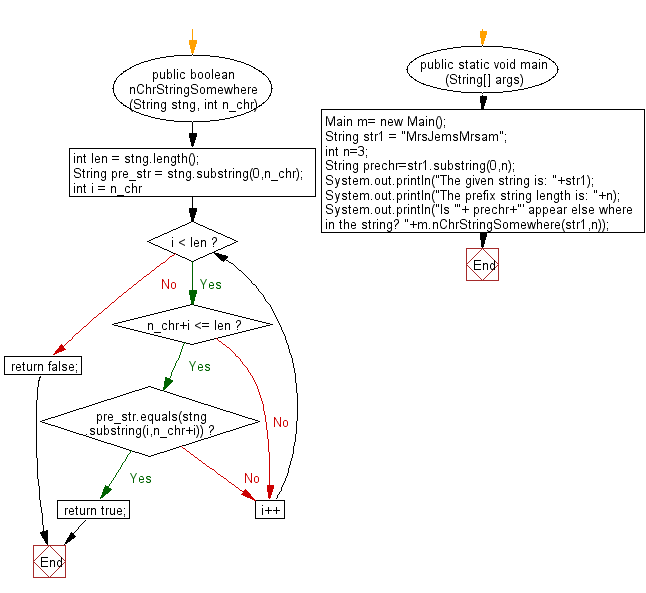﻿ Java exercises: Check whether a prefix string creates using the first specific characters in a given string appears somewhere else in the string - w3resource# Java String Exercises: Check whether a prefix string creates using the first specific characters in a given string appears somewhere else in the string

## Java String: Exercise-74 with Solution

Write a Java program to check whether a prefix string creates using the first specific characters in a given string, appears somewhere else in the string.

Sample Solution:

Java Code:

``````import java.util.*;
public class Main
{
public boolean nChrStringSomewhere (String stng, int n_chr)
{
int len = stng.length();
String pre_str = stng.substring(0,n_chr);
for (int i = n_chr; i < len; i++)
{
if(n_chr+i <= len)
{
if (pre_str.equals(stng.substring(i,n_chr+i)))
return true;
}
}
return false;
}
public static void main (String[] args)
{
Main m= new Main();
String str1 =  "MrsJemsMrsam";
int n=3;
String prechr=str1.substring(0,n);
System.out.println("The given string is: "+str1);
System.out.println("The prefix string length is: "+n);
System.out.println("Is '"+ prechr+"' appear else where in the string? "+m.nChrStringSomewhere(str1,n));
}
}
``````

Sample Output:

```The given strings is: MrsJemsmrsam
The prefix string length is: 3
Is 'Mrs' appear else where in the string? false

The given string is: MrsJemsMrsam
The prefix string length is: 3
Is 'Mrs' appear else where in the string? true
```

Pictorial Presentation:Flowchart:Java Code Editor:

Improve this sample solution and post your code through Disqus

What is the difficulty level of this exercise?

﻿

## Java: Tips of the Day

Directory Content:

Java allows you to get the names of all subdirectories and files in a folder as an array, which can then be sequentially viewed.

```import java.io.*;

public class ListContents {
public static void main(String[] args) {
File file = new File("//home//user//Documents/");
String[] files = file.list();

System.out.println("Listing contents of " + file.getPath());
for(int i=0 ; i < files.length ; i++)
{
System.out.println(files[i]);
}
}
}
```